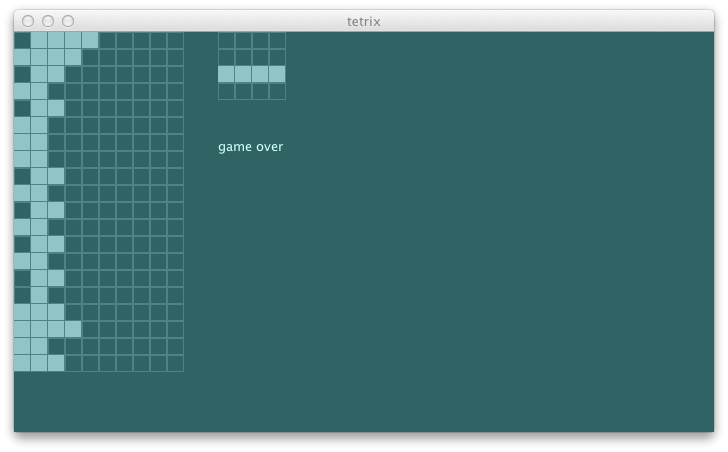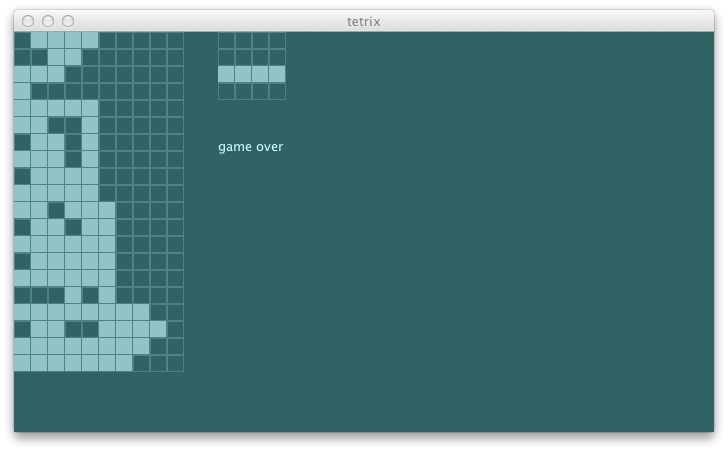# tetrix in Scala: day 7

Yesterday we started on a new challange of building tetrix-solving AI. Russell and Norvig give insight into how a rational agent can be structured using a state machine , a utility function, and a tree searching algorithm. We have the first two, and a failing test:

```[info] Solver should
[info] + pick MoveLeft for s1
[error] x pick Drop for s3
[error]    'MoveLeft' is not equal to 'Drop' (AgentSpec.scala:13)```

First we need to lay out the things we know, which is the possible moves and corresponding state transition function:

```  private[this] val possibleMoves: Seq[StageMessage] =
Seq(MoveLeft, MoveRight, RotateCW, Tick, Drop)
private[this] def toTrans(message: StageMessage): GameState => GameState =
message match {
case MoveLeft  => moveLeft
case MoveRight => moveRight
case RotateCW  => rotateCW
case Tick      => tick
case Drop      => drop
}```

To implement "What if I do action A?", use `possibleMoves`, `toTrans`, and the given state `s0` to emulate the next state. We can then use `utility` function to calculate the happiness and pick the move that maximizes the utility.

```  def bestMove(s0: GameState): StageMessage = {
var retval: StageMessage = MoveLeft
var current: Double = minUtility
possibleMoves foreach { move =>
val u = utility(toTrans(move)(s0))
if (u > current) {
current = u
retval = move
} // if
}
retval
}```

The implementation looks imperative, but it's fine as long as it's within the method. We now have the first version of the solver. To prevent the agent from cheating, we need to create a `GameMasterActor`, which issues `BestMove(s)` message to the agent actor:

```sealed trait AgentMessage
case class BestMove(s: GameState) extends AgentMessage```

Here are the actor implementations:

```class AgentActor(stageActor: ActorRef) extends Actor {
private[this] val agent = new Agent

def receive = {
case BestMove(s: GameState) =>
val message = agent.bestMove(s)
println("selected " + message)
stageActor ! message
}
}

class GameMasterActor(stateActor: ActorRef, agentActor: ActorRef) extends Actor {
def receive = {
case Tick =>
val s = getState
if (s.status != GameOver) {
agentActor ! BestMove(getState)
}
}
private[this] def getState: GameState = {
val future = (stateActor ? GetState)(1 second).mapTo[GameState]
Await.result(future, 1 second)
}
}```

This surprisingly simple yet powerful. Since the whole point of calculating the best move is to make the move, the agent actor can send it out to a `stageActor` directly. Let's hook these up:

```  private[this] val system = ActorSystem("TetrixSystem")
private[this] val stateActor = system.actorOf(Props(new StateActor(
initialState)), name = "stateActor")
private[this] val playerActor = system.actorOf(Props(new StageActor(
stateActor)), name = "playerActor")
private[this] val agentActor = system.actorOf(Props(new AgentActor(
playerActor)), name = "agentActor")
private[this] val masterActor = system.actorOf(Props(new GameMasterActor(
stateActor, agentActor)), name = "masterActor")
private[this] val tickTimer = system.scheduler.schedule(
0 millisecond, 700 millisecond, playerActor, Tick)
private[this] val masterTickTimer = system.scheduler.schedule(
0 millisecond, 700 millisecond, masterActor, Tick)```

### it's alive!

Running the swing UI, the agent actually takes over the game and starts solving tetrix!:

```[info] Running com.tetrix.swing.Main
[info] selected MoveLeft
[info] selected MoveLeft
[info] selected MoveLeft
[info] selected MoveLeft
[info] selected MoveLeft
[info] selected MoveLeft
[info] selected MoveLeft
[info] selected MoveLeft
[info] selected MoveLeft
[info] selected MoveLeft
[info] selected MoveLeft
[info] selected MoveLeft
[info] selected MoveLeft
...```And it's so dumb!

Because the search tree is too shallow it never actually reach a point where utility actually kicks in. By default it's picking `MoveLeft`. The first option is to deepen the search tree to more moves. We need eventually need that, but ultimately it's not going to solve the entire problem. First, remember, the number of nodes grows exponentially. Second, we only know about two pieces for sure.

### heuristic function

Plan B is to introduce a heuristic function.

h(n) = estimated cost of cheapest path from node n to a goal node.

Technically speaking we don't have a goal node, so the term may not apply here, but the idea is to approximate the situation to nudge tree searching to a right direction. In our case, we can think of it as having some penalty for bad shapes. For example, let's add penalty for having more than one height difference between the columns. We should square the gaps to make the penalty harsher.

```    """penalize having gaps between the columns"""          ! utility4^
...
def utility4 = {
val s = newState(Seq(
(0, 0), (0, 1), (0, 2), (0, 3), (0, 4), (0, 5), (0, 6))
map { Block(_, TKind) }, (10, 20), TKind :: TKind :: Nil)
agent.utility(s) must_== -36.0
}```

The test fails as expected:

```[info] Utility function should
[info] + evaluate initial state as 0.0,
[info] + evaluate GameOver as -1000.0,
[info] + evaluate an active state by lineCount
[error] x penalize having gaps between the columns
[error]    '0.0' is not equal to '-36.0' (AgentSpec.scala:10)```

Let's use the REPL to figure this one out. Type `console` from sbt.

```Welcome to Scala version 2.9.2 (Java HotSpot(TM) 64-Bit Server VM, Java 1.6.0_33).
Type in expressions to have them evaluated.
Type :help for more information.

scala> import com.eed3si9n.tetrix._
import com.eed3si9n.tetrix._

scala> import Stage._
import Stage._

scala> val s = newState(Seq(
(0, 0), (0, 1), (0, 2), (0, 3), (0, 4), (0, 5), (0, 6))
map { Block(_, TKind) }, (10, 20), TKind :: TKind :: Nil)
s: com.eed3si9n.tetrix.GameState = GameState(List(Block((0,0),TKind), Block((0,1),TKind), Block((0,2),TKind), Block((0,3),TKind), Block((0,4),TKind), Block((0,5),TKind), Block((0,6),TKind), Block((4,18),TKind), Block((5,18),TKind), Block((6,18),TKind), Block((5,19),TKind)),(10,20),Piece((5.0,18.0),TKind,List((-1.0,0.0), (0.0,0.0), (1.0,0.0), (0.0,1.0))),Piece((2.0,1.0),TKind,List((-1.0,0.0), (0.0,0.0), (1.0,0.0), (0.0,1.0))),List(),ActiveStatus,0)

scala> s.blocks map {_.pos} groupBy {_._1}
res0: scala.collection.immutable.Map[Int,Seq[(Int, Int)]] = Map(5 -> List((5,18), (5,19)), 4 -> List((4,18)), 6 -> List((6,18)), 0 -> List((0,0), (0,1), (0,2), (0,3), (0,4), (0,5), (0,6)))```

This is not good. We have the current piece loaded in `s.blocks`. But the `unload` is currently a private method within `Stage` object. We can refactor it out to `GameState` class as follows:

```case class GameState(blocks: Seq[Block], gridSize: (Int, Int),
currentPiece: Piece, nextPiece: Piece, kinds: Seq[PieceKind],
status: GameStatus, lineCount: Int) {
def view: GameView = ...
def unload(p: Piece): GameState = {
val currentPoss = p.current map {_.pos}
this.copy(blocks = blocks filterNot { currentPoss contains _.pos })
}
def load(p: Piece): GameState =
this.copy(blocks = blocks ++ p.current)
}```

With minor changes to `Stage` object, all tests run expect for the current one. Now REPL again:

```... the same thing as above ...

scala> s.unload(s.currentPiece)
res0: com.eed3si9n.tetrix.GameState = GameState(List(Block((0,0),TKind), Block((0,1),TKind), Block((0,2),TKind), Block((0,3),TKind), Block((0,4),TKind), Block((0,5),TKind), Block((0,6),TKind)),(10,20),Piece((5.0,18.0),TKind,List((-1.0,0.0), (0.0,0.0), (1.0,0.0), (0.0,1.0))),Piece((2.0,1.0),TKind,List((-1.0,0.0), (0.0,0.0), (1.0,0.0), (0.0,1.0))),List(),ActiveStatus,0)

scala> s.unload(s.currentPiece).blocks map {_.pos} groupBy {_._1}
res1: scala.collection.immutable.Map[Int,Seq[(Int, Int)]] = Map(0 -> List((0,0), (0,1), (0,2), (0,3), (0,4), (0,5), (0,6)))

scala> val heights = s.unload(s.currentPiece).blocks map {_.pos} groupBy {_._1} map { case (k, v) => (k, v.map({_._2}).max) }
heights: scala.collection.immutable.Map[Int,Int] = Map(0 -> 6)

scala> heights.getOrElse(1, 0)
res6: Int = 0

scala> (0 to s.gridSize._1 - 2)
res7: scala.collection.immutable.Range.Inclusive = Range(0, 1, 2, 3, 4, 5, 6, 7, 8)

scala> val gaps = (0 to s.gridSize._1 - 2).toSeq map { x => heights.getOrElse(x, 0) - heights.getOrElse(x + 1, 0) }
gaps: scala.collection.immutable.IndexedSeq[Int] = Vector(6, 0, 0, 0, 0, 0, 0, 0, 0)

scala> val gaps = (0 to s.gridSize._1 - 2).toSeq map { x => heights.getOrElse(x, 0) - heights.getOrElse(x + 1, 0) } filter {_ > 1}
gaps: scala.collection.immutable.IndexedSeq[Int] = Vector(6)

scala> gaps map {x => x * x} sum
res5: Int = 36```

I did a lot more typos and experiments than above. But you get the idea. We can incrementally construct expression using the REPL by chaining one operation after another. When we get the answer, copy-past it into the editor:

```  def utility(state: GameState): Double =
if (state.status == GameOver) minUtility
else state.lineCount.toDouble - penalty(state)
private[this] def penalty(s: GameState): Double = {
val heights = s.unload(s.currentPiece).blocks map {_.pos} groupBy {
_._1} map { case (k, v) => (k, v.size) }
val gaps = (0 to s.gridSize._1 - 2).toSeq map { x =>
heights.getOrElse(x, 0) - heights.getOrElse(x + 1, 0) } filter {_ > 1}
gaps map {x => x * x} sum
}```

The tests pass.

```[info] Utility function should
[info] + evaluate initial state as 0.0,
[info] + evaluate GameOver as -1000.0,
[info] + evaluate an active state by lineCount
[info] + penalize having gaps between the columns```

There's another problem with the current solver. Except for `Drop` the current piece is hovering midair, so it cannot be part of the evaluation. To solve this, we can simply append `Drop` unless it's already dropped. I am going to change the implementation and see which test would fail:

```  def bestMove(s0: GameState): StageMessage = {
var retval: StageMessage = MoveLeft
var current: Double = minUtility
possibleMoves foreach { move =>
val ms =
if (move == Drop) move :: Nil
else move :: Drop :: Nil
val u = utility(Function.chain(ms map {toTrans})(s0))
if (u > current) {
current = u
retval = move
} // if
}
retval
}```

Composing the transition function with `Function.chain` again. Now let's run the test.

```[info] Solver should
[info] + pick MoveLeft for s1
[error] x pick Drop for s3
[error]    'Tick' is not equal to 'Drop' (AgentSpec.scala:14)```

This is not surprising. Since we added `Drop` at the end, there's no difference between `Tick` and `Drop` anymore.
We can fix this by relaxing the spec:

```  def solver2 =
agent.bestMove(s3) must beOneOf(Drop, Tick)```

Now the agent started to pick moves other than `MoveLeft`, but it's preferring the left side of the grid a lot more.Deepening the search tree should hopefully make things better. We'll get back to this tomorrow.

```\$ git fetch origin
\$ git co day7 -b try/day7
\$ sbt "project swing" run```